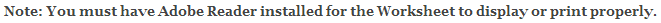# Pythagorean Theorem Worksheets

## Pythagorean Theorem Definition & Examples Worksheets

This Pythagorean Theorem Worksheet will produce five colorful and visual pages that contain definitions and Examples for the Pythagorean Theorem.

This worksheet is a great resources for the 6th Grade, 7th Grade, and 8th Grade.

Now you are ready to create your Pythagorean Theorem Worksheet by pressing the Create Button.

 Recommended Videos

If You Experience Display Problems with Your Math WorksheetClick here for More Pythagorean Theorem Worksheets